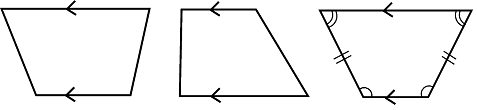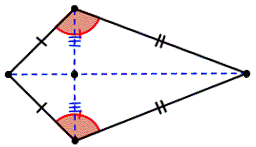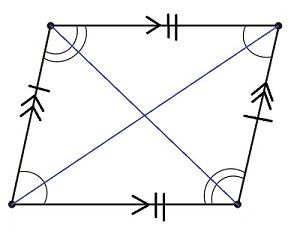# Parallelogram, Trapezium And Kite

Before talking about the types of quadrilaterals, let us recall what a quadrilateral is. A quadrilateral is a polygon which has 4 vertices and 4 sides enclosing 4 angles. The sum of its interior angles is 360 degrees. A quadrilateral, in general, has sides of different lengths and angles of different measures. However, squares, rectangles, etc. are special types of quadrilaterals with some of their sides and angles being equal. In this article, we will discuss three types of quadrilaterals – trapezium, kite, and parallelogram.

## Trapezium

A trapezium is a quadrilateral with one pair of opposite parallel sides.Properties:

• The parallel sides are called bases.
• The other two non-parallel sides are called legs.
• If the two non-parallel sides are equal and form equal angles at one of the bases, the trapezium is an isosceles trapezium.

## Kite

A kite is a quadrilateral that has 2 pairs of equal-length sides and these sides are adjacent to each other.Properties:

• The two angles are equal where the unequal sides meet.
• It can be viewed as a pair of congruent triangles with a common base.
• It has 2 diagonals that intersect each other at right angles.
• The longer or main diagonal bisects the other diagonal.
• A kite is symmetrical about its main diagonal.
• The shorter diagonal divides the kite into 2 isosceles triangles.

## Parallelogram

A parallelogram is a quadrilateral with two pairs of parallel sides.Properties:

• Opposite sides are parallel and equal in length.
• Opposite angles are equal in measure.
• Adjacent angles sum up to 180 degrees.
• It has 2 diagonals that bisect each other.
• Each diagonal divides the parallelogram into 2 congruent triangles.
• The two diagonals divide the parallelogram into 4 triangles of equal area.
• Square, rectangle, and rhombus are special types of a parallelogram.